# 基于矩阵优化填充和结构性先验统计信息的气象数据恢复Meteorological Data Restoration Based on Matrix Completion and Prior Features

DOI: 10.12677/SA.2018.72024, PDF, HTML, XML, 下载: 924  浏览: 1,224  国家自然科学基金支持

Abstract: Because of the limitation of observation means and background, combined with the complex environment, only some observation data are available. For the sake of better weather forecast, the research of meteorological data restoration based on part of observation data and matrix completion would have important scientific significance. This paper aims to, through part of real-time observation data, according to the low rank of a matrix, with applying SVT (Singular Value Thresholding) algorithm of matrix completion, obtain the deficient data so that one can make weather forecast better. The experimental result shows that the accuracy of forecast with matrix completion method is obviously higher than that with classical statistical method. When available data proportion is higher than the critical sampling proportion, errors of data filling can be controlled within 10%, which meet the requirements of meteorological data.

1. 引言

2. 矩阵填充

$\mathrm{min}\text{ }{‖X‖}_{*},\text{s}.\text{t}.\text{}{X}_{i,j}={M}_{i,j},\text{}\left(i,j\right)\in \Omega ,$ (1)

$\mathrm{min}\text{ }rank\left(X\right),\text{s}.\text{t}.\text{}{X}_{i,j}={M}_{i,j},\text{}\left(i,j\right)\in \Omega .$ (2)

$\mathrm{min}\text{ }rank\left(X\right),\text{s}.\text{t}.\text{}{P}_{\Omega }\left({X}_{i,j}\right)={P}_{\Omega }\left({M}_{i,j}\right),\text{}\left(i,j\right)\in \Omega .$ (3)

$\left\{\begin{array}{l}{X}^{k}=shrink\left({Y}^{k-1},\tau \right)\\ {Y}^{k}={Y}^{k-1}+{\delta }_{k}{P}_{\Omega }\left(M-{X}^{k}\right)\end{array}$(4)

3. 气象数据的先验特征Table 1. Historical Weather Data of Dalian (Part)

3.1. 数据的规律统计特性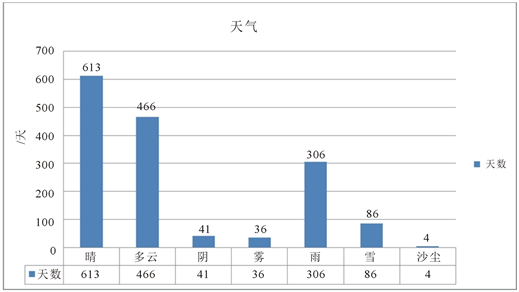Figure 1. The historical statistical weather class data of Dalian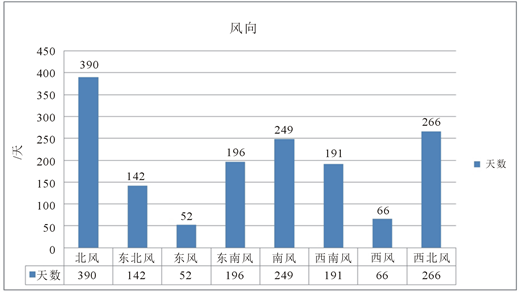Figure 2. The historical statistical wind direction data of Dalian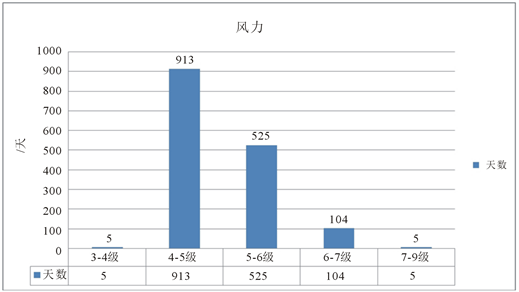Figure 3. The historical statistical wind power data of Dalian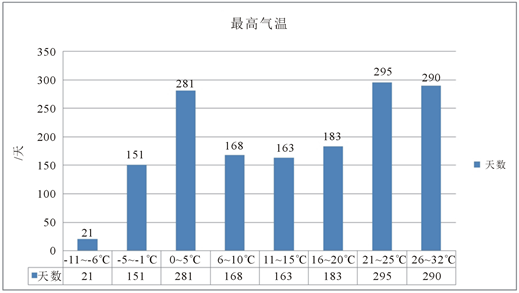Figure 4. The historical statistical highest temperature data of Dalian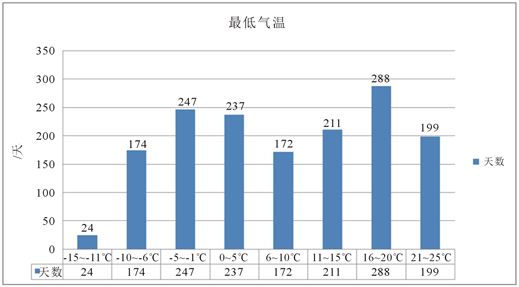Figure 5. The historical statistical lowest temperature data of Dalian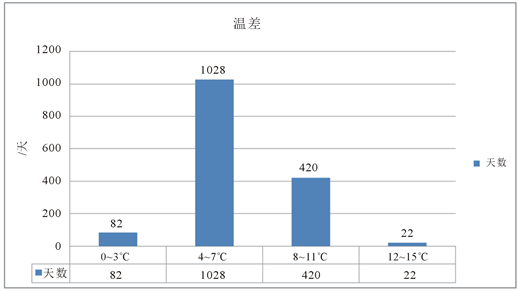Figure 6. The historical statistical temperature difference data of Dalian

3.2. 数据的分类Table 2. Historical weather data of Dalan (Part)

1) 天气类型分类

2) 风向分类

3) 风力分类

4)气温温差分类

3.3. 数据的结构性等级划分Table 3. Historical weather data after classification (Part)

1) 按月处理(表11)

1.1) 竖排结构

1.2) 横排结构

2) 按季处理(表12)

2.1) 竖排结构

2.2) 横排结构Table 4. Digitization of highest temperatureTable 5. Digitization of Lowest TemperatureTable 6. Digitization of Temperature DifferenceTable 7. Digitization of Weather ClassTable 8. Digitization of Wind Direction

4. 基于部分数据和先验特征的气象数据填充Table 10. Digitization of historical weather data of Dalian (Part)Table 11. Processing the data according to monthsTable 12. Processing the data according to seasons

5. 按月横排矩阵格式最优化分析Table 13. The error rate under different sampling rateTable 14. The error rate according to monthsTable 15. The error rate according to seasons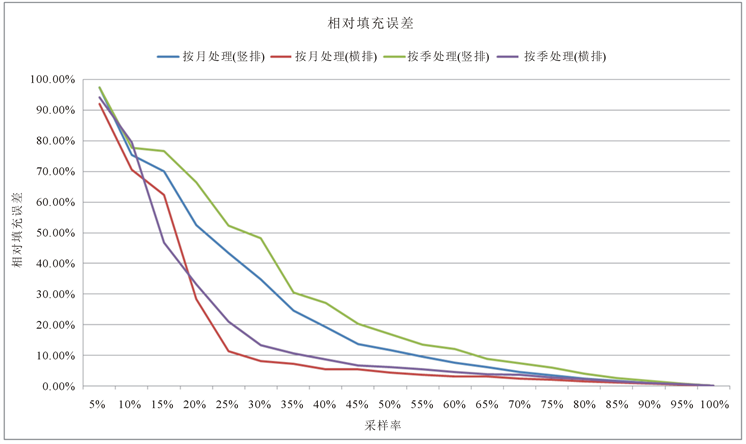Figure 7. The error rate under different sampling rate

$\xi \propto \frac{M}{m}\cdot \frac{r}{n}$(5)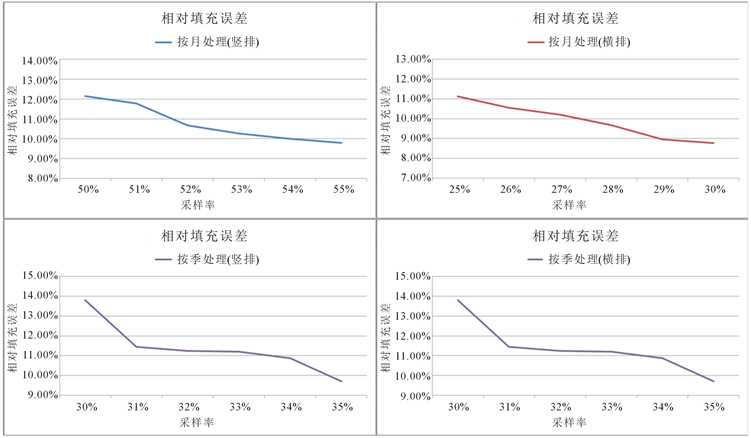Figure 8. The error rate under different sampling rate (refined)

${X}_{Tp×q}=\left[\begin{array}{l}{x}_{1,1}\text{\hspace{0.17em}}{x}_{1,2}\text{\hspace{0.17em}}\cdots \text{\hspace{0.17em}}\text{ }{x}_{1,q}\\ {x}_{2,1}\text{\hspace{0.17em}}{x}_{2,2}\text{\hspace{0.17em}}\cdots \text{\hspace{0.17em}}\text{ }{x}_{2,q}\\ ⋮\\ {x}_{p,1}\text{\hspace{0.17em}}{x}_{p,2}\text{\hspace{0.17em}}\cdots \text{\hspace{0.17em}}\text{\hspace{0.17em}}\text{\hspace{0.17em}}{x}_{p,q}\\ ⋮\\ {x}_{Tp,1}\text{\hspace{0.17em}}{x}_{Tp,2}\text{\hspace{0.17em}}\cdots \text{\hspace{0.17em}}{x}_{Tp,q}\end{array}\right]$ , (6)

${X}_{mon,}{}_{Tp×q}={X}_{Tp×q}$ , (7)

${X}_{mon,}{}_{p×Tq}=\left[\begin{array}{l}{x}_{1,1}\text{\hspace{0.17em}}{x}_{1,2}\text{\hspace{0.17em}}\cdots \text{\hspace{0.17em}}\text{ }{x}_{1,q}\text{\hspace{0.17em}}{x}_{p+1,1}\text{\hspace{0.17em}}\text{\hspace{0.17em}}\cdots \text{\hspace{0.17em}}\text{ }{x}_{\left(T-1\right)p+1,q}\\ {x}_{2,1}\text{\hspace{0.17em}}{x}_{2,2}\text{\hspace{0.17em}}\cdots \text{\hspace{0.17em}}\text{ }{x}_{2,q}\text{\hspace{0.17em}}{x}_{p+2,1}\text{\hspace{0.17em}}\text{\hspace{0.17em}}\cdots \text{\hspace{0.17em}}\text{ }{x}_{\left(T-1\right)p+2,q}\\ ⋮\text{ }\text{\hspace{0.17em}}\text{\hspace{0.17em}}\text{ }\text{ }\text{ }\text{ }\text{ }\text{ }\text{ }\text{ }\text{ }\text{\hspace{0.17em}}\text{ }⋮\\ {x}_{p,1}\text{\hspace{0.17em}}{x}_{p,2}\text{\hspace{0.17em}}\cdots \text{\hspace{0.17em}}\text{\hspace{0.17em}}\text{\hspace{0.17em}}{x}_{p,q}\text{\hspace{0.17em}}{x}_{2p,1}\text{\hspace{0.17em}}\text{\hspace{0.17em}}\cdots \text{\hspace{0.17em}}\text{ }\text{\hspace{0.17em}}\text{\hspace{0.17em}}{x}_{Tp,q}\end{array}\right]$ , (8)

${X}_{se,}{}_{Tp×q}={X}_{Tp×q}$ , (9)

${X}_{se,}{}_{p×Tq}={X}_{mon,}{}_{p×Tq}$ . (10)

${X}_{mon,}{}_{Tp×q}={U}_{mon,Tp×Tp}\left[\begin{array}{l}{\sigma }_{1}\\ \text{ }\ddots \\ \text{ }\text{ }\text{ }{\sigma }_{r1}\end{array}\right]{{V}^{\prime }}_{mon,q×q}^{}$ . (11)

6. 仿真试验Table 17. The comparison of restored measures (Average)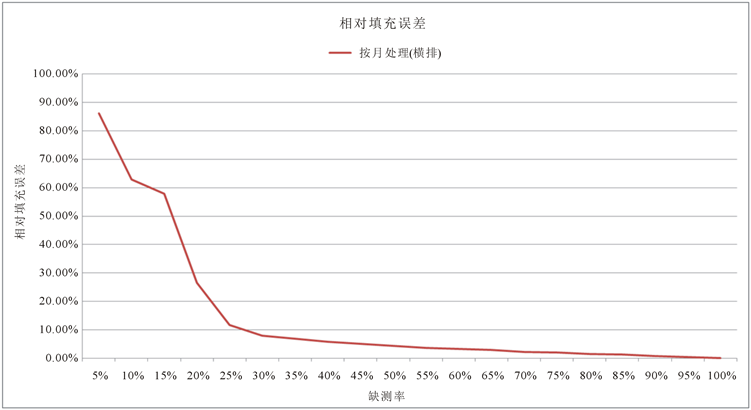Figure 9. The error rate under different sampling rate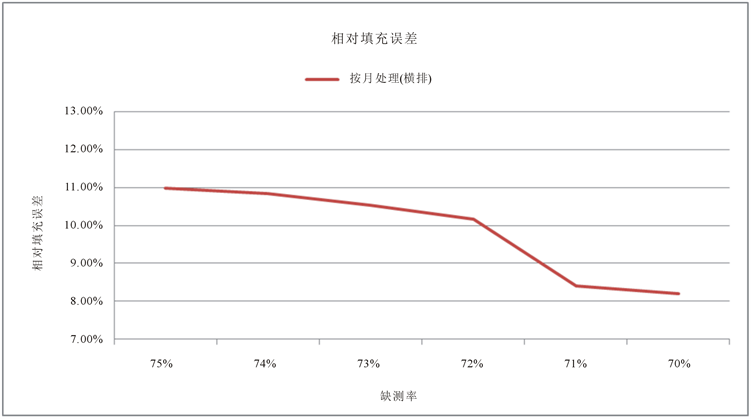Figure 10. The error rate under different sampling rate (refined)

7. 结论

NOTES

*通讯作者。

  朱乾根. 天气学原理和方法[M]. 南京: 南京气象出版社, 1992.  刘园园. 快速低秩矩阵与张量恢复的算法研究[D]: [博士学位论文]. 西安: 西安电子科技大学, 2013.  王萍, 蔡思佳, 刘宇. 基于随机投影技术的矩阵填充算法的改进[J]. 计算机应用, 2014, 34(6): 1587-1590.  Donoho, D.L. (2006) Compressed Sensing. IEEE Transactions on Information Theory, 52, 1289-1306. https://doi.org/10.1109/TIT.2006.871582  王会敏. 矩阵填充理论及其研究进展[J]. 绍兴文理学院学报, 2013, 33(7): 22-24.  黄嘉佑. 气象统计分析与预报方法[M]. 北京: 气象出版社, 2004.  王卓峥, 贾克斌. 矩阵填充与主元分析在受损图像配准中的应用[J]. 北京: 北京工业大学, 2013.  Cai, J.F., Candes, E.J. and Shen, Z.W. (2010) A Singular Value Thresholding Algorithm for Matrix Completion. SIAM Journal on Optimizatinon, 20, 1956-1982.  Ma, S., Goldfarb, D. and Chen, L. (2008) Fixed Point and Bregman Iterative Methods for Matrix Rank Minimization. Technical Report.  王永曦. 矩阵填充应用于文本分类的一些探索[D]: [硕士学位论文]. 北京: 清华大学, 2012.  郭慧杰, 赵保军. 基于矩阵填充的小波图像压缩算法[J]. 系统工程与电子技术, 2012, 34(9): 1930-1933.  孟繁驰, 李书琴, 蔡骋基. 基于核范数凸优化的微阵列缺失点重建[J]. 计算机工程与设计, 2013, 34(2): 660-664.  Keshavan, R.H., Montanari, A. and Sewoong, O.H. (2010) Matrix Completion from a Few Entries. IEEE Transactions on Information Theory, 56, 2980-2998. https://doi.org/10.1109/TIT.2010.2046205  Benjamin, R. (2009) A Simpler Approach to Matrix Completion. Journal of Machine Learning Research, 12, 3413-3430.  彭义刚, 索津莉, 戴琼海, 徐文立. 从压缩传感到低秩矩阵恢复:理论与应用[J]. 自动化学报, 2013, 39(7): 981-994.  Xu, G., Wang, X., Xu, X. and Zhou, L. (2016) Entropic Uncertainty Inequalities on Sparse Representation. IET Signal Processing, 10, 413-421. https://doi.org/10.1049/iet-spr.2014.0072  徐冠雷, 王孝通, 周立佳, 邵利民, 刘永禄, 徐晓刚. 广义测不准原理中的数学问题研究[J]. 应用数学进展, 2016, 5(3): 536-559.  Xu, G., Wang, X., Xu, X., Zhou, L. and Liu, Y. (2017) Unified Framework for Multi-Scale Decomposition and Applications. IET Journal of Engineering, 2017, 577-588.